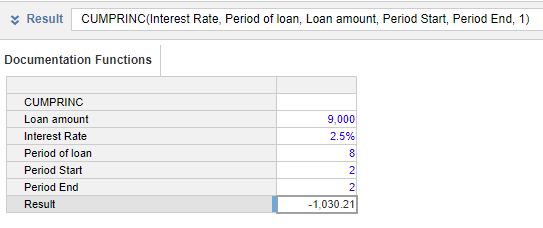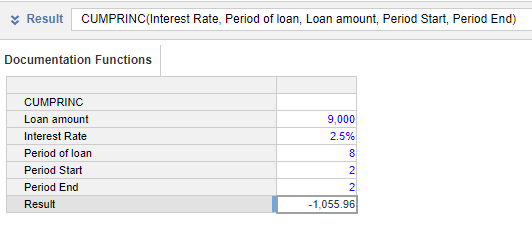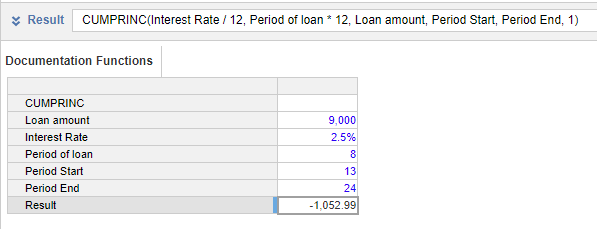1. Calculation functions
2. All Functions
3. Financial functions
4. CUMPRINCThis function calculates the cumulative total of the principal amount paid during a given period for a loan.

This function assumes equal payment amounts over the period of the loan.

The period passed in any argument in the function must be is consistent. The function takes up to six arguments: the first argument (i) requires the interest rate in the calculation period; the second (p), fourth(s), and fifth(e) arguments specify the periods used in the calculation. These periods must match, so to calculate the annual principal paid, use the annual interest rate, and the number of periods in years. Bear this in mind when using hard-coded values in the function.

This function is equivalent to the Excel function CUMPRINC.

Output

The function must be used in a line item with the Format: Number.

Syntax

CUMPRINC(i, p, v, s, e, [mode])
 Argument Description Format Available Sources i interest rate per period Number (percentage) Numeric hard-coded value, line item, or property p the number of periods over which the loan is repaid Number Numeric hard-coded value, line item, or property v the current loan balance Number Numeric hard-coded value, line item, or property s the first payment period used in the calculation. Cumulative principal amount is calculated between this point and the end period. Number Numeric hard-coded value, line item, or property e the calculation end period. Cumulative principal amount is calculated between the start period and this point. Number Numeric hard-coded value, line item, or property mode (optional) when the payments are made. If the value is not provided, or the value is zero, the function assumes payments are made at the end of each period; a non-zero value means payments are made at the start of each period. Number Numeric hard-coded value, line item, or property

Examples

The formula to calculate the principal amount paid in the second year, when payments are made at the start of the year, is:

CUMPRINC(Interest Rate, Period of loan, Loan amount, Period Start, Period End, 1)

The formula accepts the figure in the last [mode] argument and calculates the principal from the start of the period.

The line items use this data:

 Line item Value Loan amount 9000 Interest Rate 2.5 Period of loan 8 Period Start 2 Period End 2Using the same data, the formula to calculate the principal amount paid in the second year of payments, when payments are made at the end of the year, is:

CUMPRINC(Interest Rate, Period of loan, Loan amount, Period Start, Period End)The formula to calculate the total principal paid in year two, calculated monthly using periods 13 to 24 inclusive, and when payments are made at the start of the month, is:

CUMPRINC(Interest Rate/12, Period of loan*12, Loan amount, Period Start, Period End, 1)

The formula accepts the figure in the last [mode] argument and calculates the principal from the start of the period.

The line items use this data:

 Line item Value Loan amount 9000 Interest Rate 2.5 Period of loan 8 Period Start 13 Period End 24Using the same data as above, the formula to calculate the principal paid in the first month, when payments are made at the end of month, is:

CUMPRINC(Interest Rate/12, Period of loan*12, Loan amount, Period Start, Period End, 0)

Because the data is in years, the period interest rate and the number of payments over the period of the loan must be recalculated. To do this:

• divide the Interest Rate by 12, and
• multiply the Period of loan by 12.